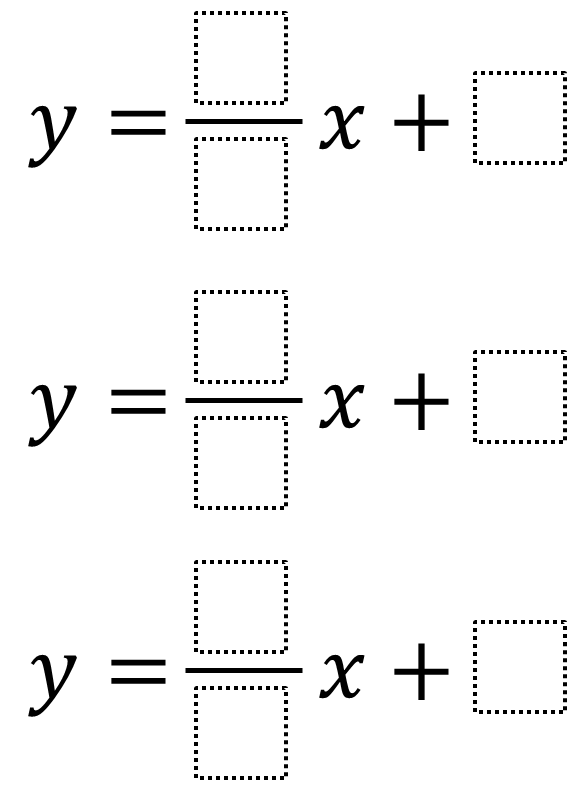Home > High School: Algebra > Reasoning with Equations and Inequalities > Systems of Equations – One Solution

# Systems of Equations – One Solution

Directions: Using the integers from -9 to 9, at most one time each, create a system of three-equations such that the solution is (1,1).### Hint

How can we determine whether a line goes through the point (1, 1)?

### Answer

One answer is y = (0/9)x + 1,  y = (-4/2)x + 3, and y= (-6/-1)x + -5.

Source: Audrey Mendivil, Daniel Luevanos, and Robert Kaplinsky

## Compound Inequalities 2

Directions: Using the digits 1 to 9, at most one time each, make two compound …